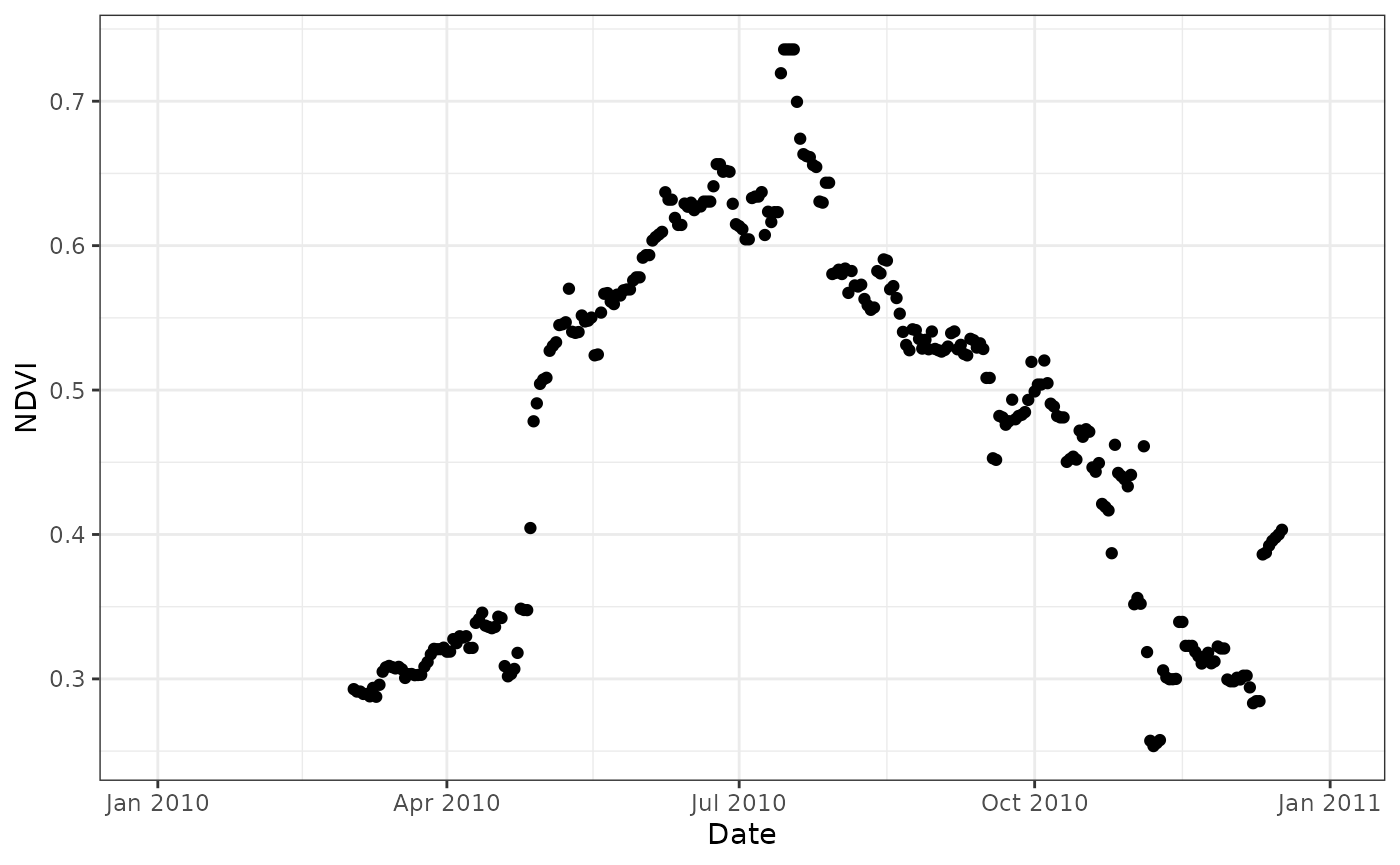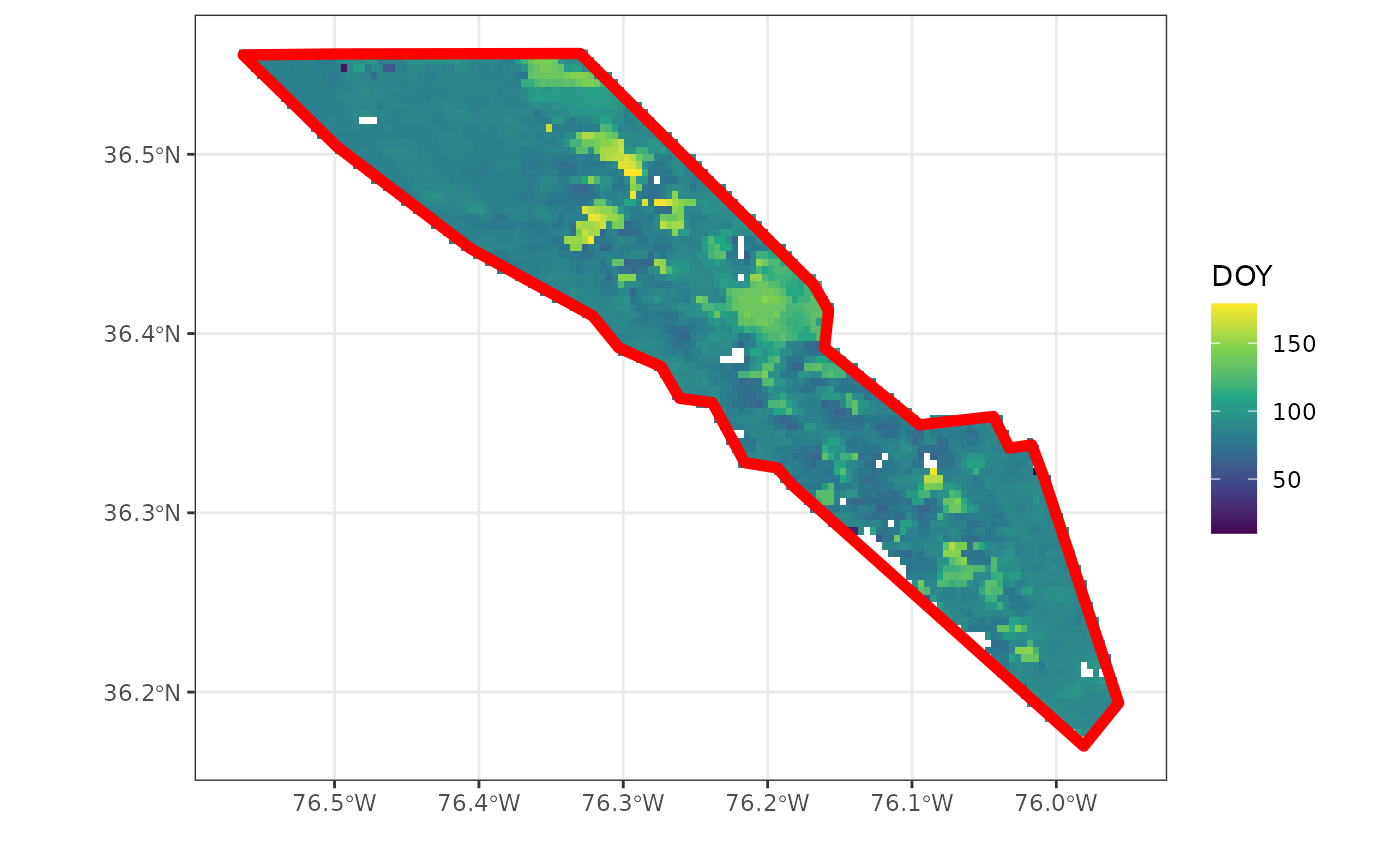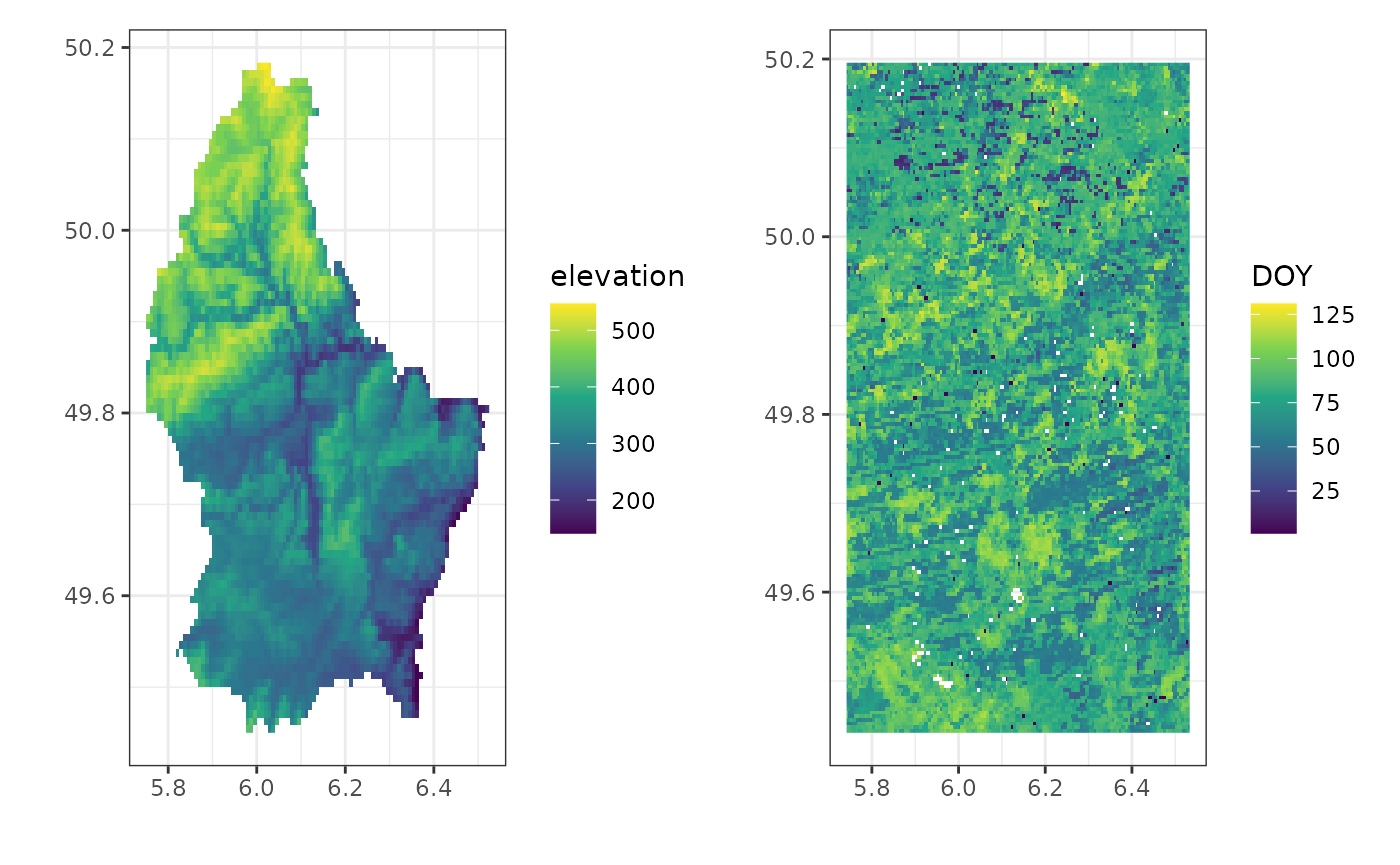## Setup

Before starting save the provided NASA Earth Data password to your local keychain. The package does not allow you to use your password inline in scripts to limit security issues when sharing scripts on github or otherwise.

``````library(appeears)

# set a key to the keychain
rs_set_key(
user = "earth_data_user",
)

# you can retrieve the password using
rs_get_key(user = "earth_data_user")

# the output should be the key you provided
# "XXXXXXXXXXXXXXXXXXXXXX"``````

Downloads are managed using a Bearer/session token. This token is valid for 48 hours, after which it will expire and you will need to request a new one. Although downloads are managed using the user (keychain) details only, you can request the current token using `rs_login()`, while `rs_logout()` will explicitly invalidate the current session token.

``````# request the current token

# invalidate the current session
rs_logout(token)``````

## Use

### Listing available data

You can list all available products on the AppEEARS API using the `rs_products()` function. Similarly, you can list all available layers for a given product using `rs_layers()`. Note that when queryign the layers for a product you will need to use the field `ProductAndVersion` field, as some products are listed under various versions.

``````# list all product information
products <- rs_products()

#>  "MCD12Q1.061"               "MCD12Q2.061"

# list all layers for a particular
# product
layers <- rs_layers(
product = "MCD12Q2.006"
)

#>                  V1    Band

### Point based data requests

All point based queries are made by first creating a tidy data frame with the desired products and layers to query.

In this data frame `task` specifies the overall name of the task to run (this prefix will be used to name the final downloaded files). The `subtask` denotes the various locations and or products you want to query. As such, you can query multiple locations in the same larger task, avoiding multiple queries to the API.

The `latitude` and `longitude` fields specify geographic coordinates of query locations, while `start` and `end` columns define the range of the data queried. Note that the date range will cover the maximum date range across all `subtasks`. If date ranges vary widely it is advised to create separate tasks.

Finally the `product` and `layer` columns denote the remote sensing product and particular layer to download. A full list of products can be queried using `rs_products()`, while the layers of a particular product can be listed using `rs_layers()`. Note that the product needs to be specified using the full product name, including the version of the product (as stored in the `ProductAndVersion` field).

For point and area based queries all data are saved in a subdirectory of the main `path` as defined by the task name.

``````# Load the library
library(appeears)

rs_products()

# list layers of the MOD11A2.061 product
rs_layers("MOD11A2.061")

df <- data.frame(
latitude = 42.5378,
longitude = -72.1715,
start = "2010-01-01",
end = "2010-12-31",
product = "MCD43A4.061",
)

# build the area based request/task
# rename the task name so data will
# be saved in the "point" folder
# as defined by the task name

# request the task to be executed
rs_request(
user = "earth_data_user",
transfer = TRUE,
path = "~/some_path",
verbose = TRUE
)``````

``````# read in data
"~/some_path/time_series/time-series-MCD43A4-061-results.csv",
sep = ","
)``````

The downloaded data contains both requested bands as columns and we can do band math easily. Below the Normalized Difference Vegetation Index (NDVI) is calculated using bands 3 (red) and band 4 (NIR). We use the MODLAND quality control bands to screen out spurious values (those equal to 255). Finally, a quick plot is generated for the NDVI values for year 2010.

``````# convert band 3 and 4 to NDVI
time_series <- time_series |>
mutate(
Date = as.Date(Date),
)

# screen for quality control
time_series <- time_series |>
mutate(
NDVI = ifelse(MCD43A4_061_BRDF_Albedo_Band_Mandatory_Quality_Band4 == 255 |
MCD43A4_061_BRDF_Albedo_Band_Mandatory_Quality_Band3 == 255,
NA, NDVI)
)

# plot the time series
ggplot(time_series) +
geom_point(
aes(
Date,
NDVI
)
) +
theme_bw()``````### Area based data requests

You can select a region-of-interest (ROI) instead of point based data, using both `sf` polygons or the extent (bounding box) of an existing `terra` `SpatRaster` object. Both methods follow the same workflow.

#### {sf} polygon ROI

When using an `sf` object, provide it to the `roi` argument of the `rs_build_task()` function. The `sf` object must be of class `sf` not `sfc` when required convert `sfc` data using `st_as_sf()`.

Note however that at the time only as simple polygon is supported. Multiple polygons in the same `sf` object might result in failure to query the data.

Furthermore, no other means will be provided to specify a region-of-interest. As such, you will always have to query a region-of-interest using an `sf` object. This ensures consistency across queries and allows for rapid visualization of a region of interest (in contrast to a simple list of e.g. top-left, bottom-right coordinates).

``````# load the required libraries
library(appeears)
library(sf)
library(dplyr)
library(ggplot2)

df <- data.frame(
latitude = 42.5378,
longitude = -72.1715,
start = "2010-01-01",
end = "2010-12-31",
product = "MCD12Q2.006",
layer = c("Greenup")
)

# load the north carolina demo data
# included in the {sf} package
# and only retain Camden county
roi <- st_read(system.file("gpkg/nc.gpkg", package="sf"), quiet = TRUE) |>
filter(
NAME == "Camden"
)``````
``````# build the area based request/task
# rename the task name so data will
# be saved in the "polygon" folder
# as defined by the task name
df = df,
roi = roi,
format = "geotiff"
)

# request the task to be executed
rs_request(
user = "earth_data_user",
transfer = TRUE,
path = "~/some_path",
verbose = TRUE
)``````

Once downloaded the geotiff can be read in using the `terra` package from the subfolder defined by the `task` name.

``````library(terra)
r_polygon <- terra::rast(
file.path("~/some_path","polygon/MCD12Q2.006_Greenup_0_doy2010001_aid0001.tif")
)``````

A quick plot of both the raster and vector polygon show the cut-out as provided by the AppEEARS API.

``````# convert to data frame for plotting
# with ggplot2, otherwise use the
# tidyterra package and geom_spatrast()
df_polygon <- r_polygon |>
as.data.frame(xy=TRUE)

# convert incremental values (days since Jan 1, 1970
# to DOY)
df_polygon <- df_polygon |>
mutate(
DOY =  as.numeric(
format(
as.Date("1970-01-01") + MCD12Q2.006_Greenup_0_doy2010001_aid0001, "%j"
)
)
) |>
filter(
DOY < 180
)

#>           x        y MCD12Q2.006_Greenup_0_doy2010001_aid0001 DOY
#> 1 -76.56458 36.55625                                    14695  86
#> 2 -76.56042 36.55625                                    14692  83
#> 3 -76.55625 36.55625                                    14692  83
#> 4 -76.55208 36.55625                                    14692  83
#> 5 -76.54792 36.55625                                    14688  79
#> 6 -76.54375 36.55625                                    14690  81

ggplot() +
geom_raster(
data = df_polygon,
aes(
x,
y,
fill = DOY
)
) +
labs(
x = "",
y = ""
) +
scale_fill_viridis_c() +
geom_sf(
data = roi,
fill = NA,
colour = "red",
lwd = 2
) +
theme_bw()``````#### {terra} SpatRaster ROI

The `terra` based region-of-interest workflow is similar to that of `sf` polygon based queries. One only has to provide a `SpatRaster` as an `roi` argument in `rs_build_task()` to query a region of the same extent as the `SpatRaster`. The use case for this functionality is obvious, creating a quick way to sample new data for an existing data set (using the same coverage).

Note that unlike the `sf` method a bounding box is used and masked data is ignored (the full extent is downloaded and masking will have to be repeated afterwards).

``````# load the required libraries
library(terra)
library(ggplot2)
library(patchwork)

# create a SpatRaster ROI from the terra demo file
f <- system.file("ex/elev.tif", package="terra")
roi <- terra::rast(f)``````
``````
# build the area based request/task
# rename the task name so data will
# be saved in the "raster" folder
# as defined by the task name
df = df,
roi = roi,
format = "geotiff"
)

# request the task to be executed
rs_request(
user = "earth_data_user",
transfer = TRUE,
path = "~/some_path",
verbose = TRUE
)``````

Once downloaded the geotiff can be read in using the `terra` package from the subfolder defined by the `task` name.

``````r_raster <- terra::rast(
file.path("~/some_path","raster/MCD12Q2.006_Greenup_0_doy2010001_aid0001.tif")
)``````

A quick plot of both the raster and vector polygon show the cut-out as provided by the AppEEARS API.

``````# convert to data frame for plotting
# with ggplot2, otherwise use the
# tidyterra package and geom_spatrast()

df_raster <- r_raster |>
as.data.frame(xy=TRUE)

# convert incremental values (days since Jan 1, 1970
# to DOY)
df_raster <- df_raster |>
mutate(
DOY =  as.numeric(
format(
as.Date("1970-01-01") + MCD12Q2.006_Greenup_0_doy2010001_aid0001, "%j"
)
)
) |>
filter(
DOY < 180
)

df_source <- roi |>
as.data.frame(xy=TRUE)

p <- ggplot() +
geom_raster(
data = df_source,
aes(
x,
y,
fill = elevation
)
) +
labs(
x = "",
y = ""
) +
scale_fill_viridis_c() +
theme_bw()

p2 <- ggplot() +
geom_raster(
data = df_raster,
aes(
x,
y,
fill = DOY
)
) +
labs(
x = "",
y = ""
) +
scale_fill_viridis_c() +
theme_bw()

# patchwork side by side plot
p | p2``````